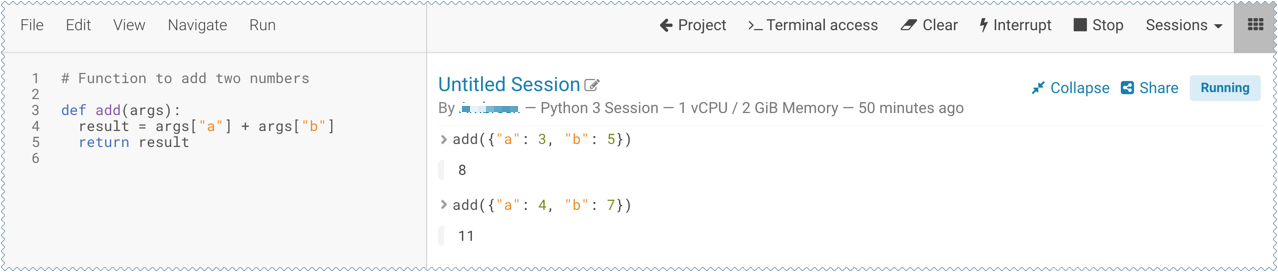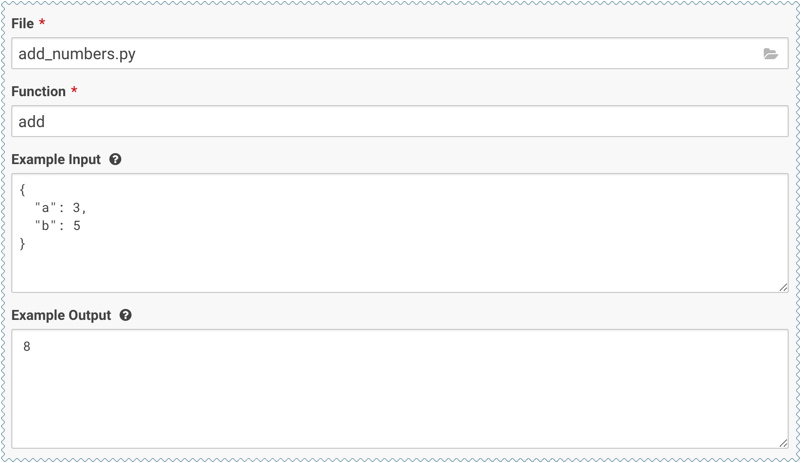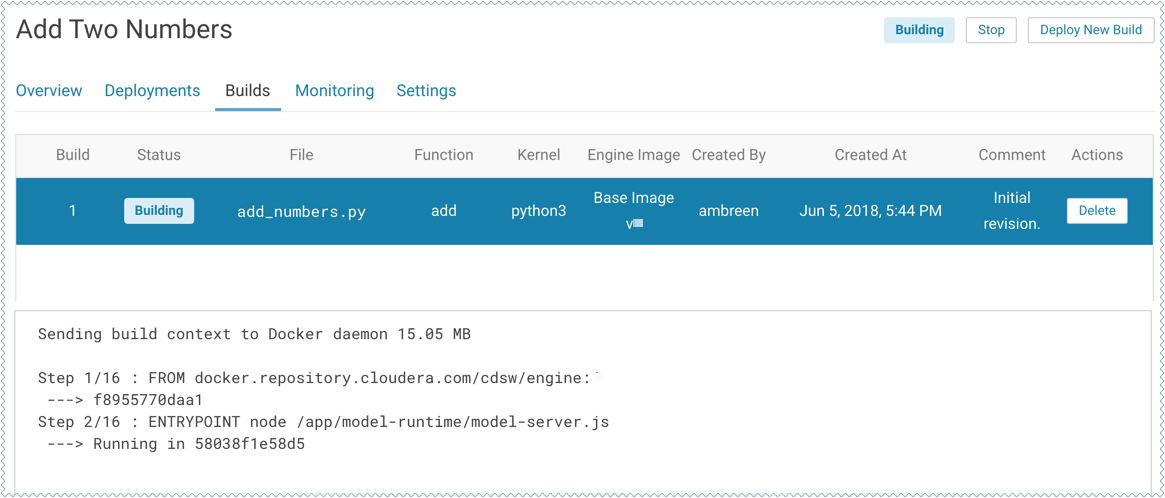# Creating and Deploying a Model (QuickStart)

Using Cloudera Data Science Workbench, you can create any function within a script and deploy it to a REST API. In a machine learning project, this will typically be a predict function that will accept an input and return a prediction based on the model's parameters.

For the purpose of this quick start demo we are going to create a very simple function that adds two numbers and deploy it as a model that returns the sum of the numbers. This function will accept two numbers in JSON format as input and return the sum.
1. Create a new project. Note that models are always created within the context of a project.
2. Click Open Workbench and launch a new Python 3 session.
3. Create a new file within the project called `add_numbers.py`. This is the file where we define the function that will be called when the model is run. For example:
``````def add(args):
result = args["a"] + args["b"]
return result``````
4. Before deploying the model, test it by running the `add_numbers.py` script, and then calling the `add` function directly from the interactive workbench session. For example:
``add({"a": 3, "b": 5})``5. Deploy the `add` function to a REST endpoint.
1. Go to the project Overview page.
2. Click Models > New Model.
3. Give the model a Name and Description.
4. Enter details about the model that you want to build. In this case: